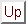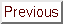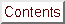Next: Cholesky decomposition. Up: Annexes Previous: 1D Newton's search   Contents

# Newton's method for non-linear equations

We want to find the solutionof the set of non-linear equations: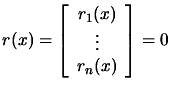(13.31)

The algorithm is the following:
1. Choose2. Calculate a solution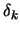to the Newton equation:(13.32)

3.We use a linear model to derive the Newton step (rather than a quadratical model as in unconstrained optimization) because the linear model normally as a solution and yields an algorithm with fast convergence properties (Newton's method has superlinear convergence when the Jacobian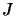is a continuous function and local quadratical convergence whenis Liptschitz continous). Newton's method for unconstrained optimization can be derived by applying Equation 13.32 to the set of nonlinear equations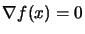).

Frank Vanden Berghen 2004-04-19